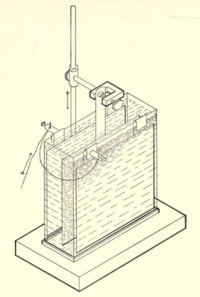# Copper coulometer

﻿
Copper coulometerCopper coulometer

The copper coulometer is a one of the common application of the copper-copper(II) sulfate electrode. Such a coulometer consists of two identical copper electrodes immersed in slightly acidic pH-buffered solution of copper(II) sulfate. Passing of current through the element leads to the anodic dissolution of the metal on anode and simultaneous deposition of copper-ions on the cathode. These reactions have 100% efficiency over a wide range of the current densities.

## Calculation

Amount of the quantity of electricity passed through the cell can be easily calculated by mass changes of any of the electrodes:$Q = \frac{ \Delta\ m*2*F}{63.546}$,

where:

• Q is the quantity of electricity (coulombs)
•$\Delta\ m$ is the mass transported (gm)
• 63.546 is the atomic weight of copper (the factor 2 is due to the transport of divalent ions)
• F is the Faraday constant (96485.3383)

Although from a theoretical and historical point of view this apparatus is interesting, present day electronic measurement of time and electrical current provide in their multiplication the amount of passed coulombs much easier, with greater precission, and in a shorter period of time than is possible by weighing the electrodes.